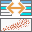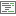# Generalized Linear RegressionPerforms Generalized Linear Regression (GLR) to generate predictions or to model a dependent variable in terms of its relationship to a set of explanatory variables. This tool can be used to fit continuous (Gaussian), binary (logistic), and count (Poisson) models.

## Analysis using GeoAnalytics Tools

Analysis using GeoAnalytics Tools is run using distributed processing across multiple ArcGIS GeoAnalytics Server machines and cores. GeoAnalytics Tools and standard feature analysis tools in ArcGIS Enterprise have different parameters and capabilities. To learn more about these differences, see Feature analysis tool differences.

## Examples

• As a GIS analyst at a utility company, you have a dataset of power outages, as well as extreme weather data. You enrich your outage data using the Build Multi-Variable Grid and Enrich from Multi-Variable Grid tools to create a dataset with extreme weather information for the outages. You use Generalized Linear Regression to determine what event led to the power outages. Now that you have this information, you can predict outages and allocate resources.
• As an analyst for a large city, you have historic 911 call records, as well as demographic information. You need to answer the following questions: Which variables effectively predict 911 call volume? Given future projections, what is the expected demand for emergency response resources?

## Usage notes

This tool can be used in two operation modes. The Fit a model to assess model performance option can be used to evaluate the performance of different models as you explore different explanatory variables and tool settings. Once a good model has been found, you can use the Fit a model and predict values option.

Use the Choose a layer to generate a model from parameter with a field representing the phenomena you are modeling (Choose the field to model) and one or more fields representing the explanatory variables. These fields must be numeric and have a range of values. Features that contain missing values in the dependent or explanatory variable will be excluded from the analysis. If you want to modify null values, use the Calculate Field tool first to create a new layer with updated values.

The Generalized Linear Regression tool also produces output features and diagnostics. Output feature layers are automatically added to the map with a rendering scheme applied to model residuals. A full explanation of each output is provided below.

It is important to use the correct model (Continuous, Binary, or Count) for your analysis to obtain accurate results of your regression analysis.

Model summary results and diagnostics are written to the messages window and charts will be created below the output feature class. The diagnostics reported depend on the Model Type. The three options for model type are as follows:

• Use the Continuous (Gaussian) model type if your dependent variable can take on a wide range of values such as temperature or total sales. Ideally, your dependent variable will be normally distributed.
• Use a Binary (logistic) model type if your dependent variable can take on one of two possible values, such as success and failure or presence and absence. The field containing your dependent variable must be numeric and contain only ones and zeros. There must be variation of the ones and zeros in your data.

• Consider using a Count (Poisson) model type if your dependent variable is discrete and represents the number of occurrences of an event such as a count of crimes. Count models can also be used if your dependent variable represents a rate and the denominator of the rate is a fixed value such as sales per month or number of people with cancer per 10,000 in the population. A Count model assumes that the mean and variance of the dependent variable are equal, and the values of your dependent variable cannot be negative or contain decimals.

The dependent variable and explanatory variable parameters should be numeric fields containing a range of values. This tool cannot solve when variables have the same values (if all the values for a field are 9.0, for example).

Features with one or more null values or empty string values in prediction or explanatory fields will be excluded from the output. If needed, you can modify values using Calculate Field.

You should visually inspect the over- and underpredictions evident in your regression residuals to see if they provide clues about potential missing variables from your regression model.

You can use the regression model that has been created to make predictions for other features. Creating these predictions requires that each prediction feature(Choose a layer to predict values for) has values for each of the explanatory variables provided. If the field names from the input features and prediction locations parameters do not match, a variable matching parameter is provided. When matching the explanatory variables, the fields from the input features and prediction locations parameters must be of the same type (double fields must be matched with double fields, for example).

## Outputs

The Generalized Linear Regression tool produces a variety of outputs. A summary of the GLR model and statistical summaries are available on the portal item page and as a resource on your layer. To access the summary of your results, click Show Resultsunder your resulting layer in Map Viewer. The tool generates at least one output layer and an optional output predicted features. The output features are automatically added to Map Viewer with a hot and cold rendering scheme applied to model residuals. The diagnostics generated depend on the model type of the input features and are described below.

### Continuous (Gaussian)

#### Interpret messages and diagnostics

• AIC—This is a measure of model performance and can be used to compare regression models. Taking into account model complexity, the model with the lower AIC value provides a better fit to the observed data. AIC is not an absolute measure of goodness of fit but is useful for comparing models with different explanatory variables as long as they apply to the same dependent variable. If the AIC values for two models differ by more than 3, the model with the lower AIC value is considered more accurate.
• AICc—AICc applies a bias correction to AIC for small sample sizes. AICc will approach AIC as the number of features in the input increase. See AIC above.
• Multiple R-Squared—The R-Squared is a measure of goodness of fit. Its value varies from 0.0 to 1.0, with higher values being preferable. It may be interpreted as the proportion of dependent variable variance accounted for by the regression model. The denominator for the R-Squared computation is the sum of squared dependent variable values. Adding an extra explanatory variable to the model does not alter the denominator but does alter the numerator; this gives the impression of improvement in model fit that may not be real. See Adjusted R-Squared below.
• Adjusted R-Squared—Because of the problem described above for the R-Squared value, calculations for the adjusted R-Squared value normalize the numerator and denominator by their degrees of freedom. This has the effect of compensating for the number of variables in a model, and consequently, the Adjusted R-Squared value is almost always less than the R-Squared value. However, in making this adjustment, you lose the interpretation of the value as a proportion of the variance explained. In Geographically Weighted Regression (GWR), the effective number of degrees of freedom is a function of the neighborhood used, so the adjustment may be quite marked in comparison to a global model such as GLR. For this reason, AICc is preferred as a means of comparing models.

### Binary (Logistic)

#### Interpret messages and diagnostics

• AIC—This is a measure of model performance and can be used to compare regression models. Taking into account model complexity, the model with the lower AIC value provides a better fit to the observed data. AIC is not an absolute measure of goodness of fit but is useful for comparing models with different explanatory variables as long as they apply to the same dependent variable. If the AIC values for two models differ by more than 3, the model with the lower AIC value is considered more accurate.
• AICc—AICc applies a bias correction to AIC for small sample sizes. AICc will approach AIC as the number of features in the input increase. See AIC above.

### Count (Poisson)

#### Interpret messages and diagnostics

• AIC—This is a measure of model performance and can be used to compare regression models. Taking into account model complexity, the model with the lower AIC value provides a better fit to the observed data. AIC is not an absolute measure of goodness of fit but is useful for comparing models with different explanatory variables, as long as they apply to the same dependent variable. If the AIC values for two models differ by more than 3, the model with the lower AIC value is considered more accurate.
• AICc—AICc applies a bias correction to AIC for small sample sizes. AICc will approach AIC as the number of features in the input increase. See AIC above.

## Limitations

The GeoAnalytics implementation of Generalized Linear Regression has the following limitations:

• It is a global regression model and does not take the spatial distribution of data into account.
• Analysis does not apply Moran's I test on the residuals.
• Feature datasets (points, lines, polygons and tables) are supported as input; rasters are not supported.
• You cannot classify values into multiple classes.

## ArcGIS API for Python example

The Generalized Linear Regression tool is available through ArcGIS API for Python.

This example fits a model on a dataset and applies the prediction to another.

``````# Import the required ArcGIS API for Python modules
import arcgis
from arcgis.gis import GIS

# Connect to your ArcGIS Enterprise portal and check that GeoAnalytics is supported
portal = GIS("https://myportal.domain.com/portal", "gis_publisher", "my_password", verify_cert=False)
if not portal.geoanalytics.is_supported():
print("Quitting, GeoAnalytics is not supported")
exit(1)

# Search for and list the big data file shares in your portal
search_result = portal.content.search("", "Big Data File Share")

# Look through search results for a big data file share with the matching name that you want to model
model_layer = next(x for x in search_result if x.title == "bigDataFileShares_Sales_2018")

# Find the dataset you want to predict
predict_layer = portal.content.search("Sales_2025", "Feature Layer")
predict_layer = layer_result.layers

# Run the tool Generalized Linear Regression
glr_result = arcgis.geoanalytics.analyze_patterns.glr(input_layer = model_layer,
features_to_predict = predict_layer,
var_dependent = "chicago_crimes_enriched",
regression_family = "Count",
exp_var_matching = [{"predictionLayerField":"store_count", "trainingLayerField": "num_of_stores"}],
output_name = "predicted_customers")

# Visualize the results if you are running Python in a Jupyter Notebook
processed_map = portal.map()
processed_map``````

## Similar tools

Use the ArcGIS GeoAnalytics Server Generalized Linear Regression tool to generate predictions or to model a dependent variable in terms of its relationship to a set of explanatory variables. Other tools may be useful in solving similar but slightly different problems.

### Map Viewer analysis tools

Create models and predictions using the ArcGIS GeoAnalytics Server Forest-based Classification and Regression tool.

### ArcGIS Desktop analysis tools

To run this tool from ArcGIS Pro, your active portal must be Enterprise 10.7 or later. You must sign in using an account that has privileges to perform GeoAnalytics Feature Analysis.

Perform similar regression operations in ArcGIS Pro with the Generalized Linear Regression geoprocessing tool as part of the Spatial Statistics toolbox.

Create models and predictions using an adaptation of Leo Breiman's random forest algorithm in ArcGIS Pro with the Forest-based Classification and Regression geoprocessing tool as part of the Spatial Statistics toolbox.

Preform GWR in ArcGIS Pro with the Geographically Weighted Regression geoprocessing tool as part of the Spatial Statistics toolbox.